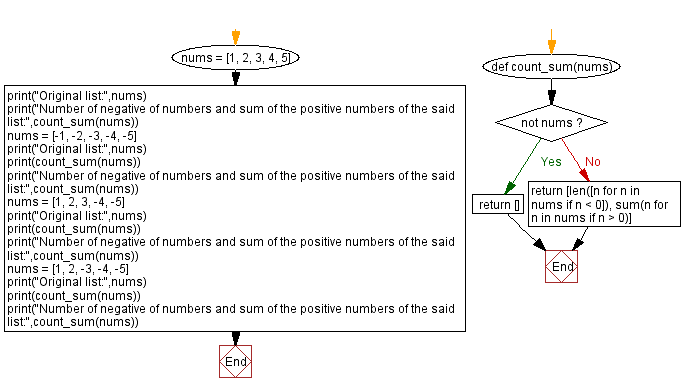﻿ Python: Count negative numbers and sum of positive numbers of a given list - w3resource

# Python: Count negative numbers and sum of positive numbers of a given list

## Python Basic - 1: Exercise-84 with Solution

Write a Python program that accepts a list of numbers. Count the negative numbers and compute the sum of the positive numbers of the said list. Return these values through a list.

Sample Solution:

Python Code:

``````def count_sum(nums):
if not nums: return []
return [len([n for n in nums if n < 0]), sum(n for n in nums if n > 0)]
nums = [1, 2, 3, 4, 5]
print("Original list:",nums)
print("Number of negative of numbers and sum of the positive numbers of the said list:",count_sum(nums))
nums = [-1, -2, -3, -4, -5]
print("Original list:",nums)
print(count_sum(nums))
print("Number of negative of numbers and sum of the positive numbers of the said list:",count_sum(nums))
nums = [1, 2, 3, -4, -5]
print("Original list:",nums)
print(count_sum(nums))
print("Number of negative of numbers and sum of the positive numbers of the said list:",count_sum(nums))
nums = [1, 2, -3, -4, -5]
print("Original list:",nums)
print(count_sum(nums))
print("Number of negative of numbers and sum of the positive numbers of the said list:",count_sum(nums))
``````

Sample Output:

```Original list: [1, 2, 3, 4, 5]
Number of negative of numbers and sum of the positive numbers of the said list: [0, 15]
Original list: [-1, -2, -3, -4, -5]
[5, 0]
Number of negative of numbers and sum of the positive numbers of the said list: [5, 0]
Original list: [1, 2, 3, -4, -5]
[2, 6]
Number of negative of numbers and sum of the positive numbers of the said list: [2, 6]
Original list: [1, 2, -3, -4, -5]
[3, 3]
Number of negative of numbers and sum of the positive numbers of the said list: [3, 3]
```

Pictorial Presentation:Flowchart:Python Code Editor:

Have another way to solve this solution? Contribute your code (and comments) through Disqus.

What is the difficulty level of this exercise?

Test your Programming skills with w3resource's quiz.

﻿